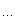# 那谁的技术博客

## 在一个有序序列中查找重复/不存在的数

<= right时继续循环:
pos
= (left + right) / 2

如果 序列的第pos个元素不等于pos
那么不存在的元素一定出现在pos之前, 将right
= pos - 1
否则
pos之前的元素都是存在的, 因此 left
= pos + 1

1) 二分查找算法(迭代和递归版本)
2) 求出不在里面的数来

C代码如下:
/*
* 查找有序序列中 重复/不存在 的数算法演示
* 版权所有:
http://www.cppblog.com/converse/

*/

#include
<stdio.h>
#include
<stdlib.h>
#include
<time.h>

#define FUNC_IN()   printf("\nin  %s\n\n", __FUNCTION__)
#define FUNC_OUT()  printf("\nout %s\n\n", __FUNCTION__)

/* 生成一个长度为len的数组, 其中array[i] = i(0<=i<len) */
void generate_array(int array[], int len);

/* 向长度为len的有序数组中新增一个元素x(0<=x<len - 1), 同时保持原有数组有序 */
void add_to_array(int array[], int len, int x);

/* 删除长度为len的有序数组中的一个元素x(0<=x<len), 同时保存原有数组有序 */
void del_from_array(int array[], int len, int x);

/* 打印一个数组 */
void display_array(int array[], int len);

/* 查找一个长度为len的有序数组中哪个元素不存在 */
int find_not_exist(int array[], int len);

/* 查找一个长度为len的有序数组中哪个元素重复了 */
int find_duplicate(int array[], int len);

int main(int argc, char *argv[])
{

int count = 10;

int *array = NULL;

srand(time(NULL));

if (argc == 2)
{
count
= atoi(argv);
}

/* 申请内存的时候多申请一个元素 */

if ((array = (int *)malloc((count + 1* sizeof(int))) == NULL)
{
printf(
"malloc error!\n");
exit(
-1);
}

/* 首先生成一个元素都是有序的整型数组 */
generate_array(array, count);

display_array(array, count);

/* 删除其中的一个元素 */
del_from_array(array, count, rand()
% count);

display_array(array, count);

/* 查找不存在的元素 */

int x = find_not_exist(array, count);
printf(
"the element not exist is %d\n", x);

/* 把删除的元素补上 */
+ 1, x);

/* 新增一个重复的元素 */
+ 1, rand() % count);

display_array(array, count
+ 1);

/* 查找重复的元素 */
printf(
"the element duplicate is %d\n", find_duplicate(array, count + 1));

free(array);

return 0;
}

void generate_array(int array[], int len)
{

int i;

for (i = 0; i < len; ++i)
{
array[i]
= i;
}
}

void add_to_array(int array[], int len, int x)
{

int i;

/* 把[x + 1, len - 1]之间的元素右移一个位置 */

for (i = x + 1; i < len - 1++i)
{
array[i
+ 1= i;
}

/* x + 1的位置保存重复的x, 这样数组仍然是有序的 */
array[x
+ 1= x;
}

void del_from_array(int array[], int len, int x)
{

int i;

/* 将[x, len)的元素向左移动一个位置 */

for (i = x; i < len; ++i)
{
array[i]
= i + 1;
}

printf(
"del_from_array element is %d\n", x);
}

void display_array(int array[], int len)
{

int i;

printf(
"\n{ ");

for (i = 0; i < len; ++i)
{
printf(
"%d ", array[i]);
}

printf(
"}\n");
}

int find_not_exist(int array[], int len)
{
FUNC_IN();

int left, right, pos, count;

for (left = 0, right = len - 1, count = 1; left <= right; count++)
{
pos
= (left + right) / 2;

printf(
"[%d] left = %d, pos = %d, right = %d\n", count, left, pos, right);

/*
* 如果array[pos] != pos, 那么不存在的元素一定在[left, pos - 1]之间

*/

if (array[pos] != pos)
{
right
= pos - 1;
}

else
{
left
= pos + 1;
}
}

FUNC_OUT();

/* left之前的元素都是存在的 */

return left;
}

int find_duplicate(int array[], int len)
{

/*
* find_not_exist()函数的返回值表示在它之前的元素都是正确的,
* 因此这个返回位置就是有重复的元素位置 + 1, 于是要减去1
*
*/

return find_not_exist(array, len) - 1;
}

posted on 2009-08-23 20:24 那谁 阅读(4542) 评论(3)  编辑 收藏 引用 所属分类: 算法与数据结构## #re: 在一个有序序列中查找重复/不存在的数  回复更多评论

2009-08-24 12:20 | 阿福

## #re: 在一个有序序列中查找重复/不存在的数  回复更多评论

@阿福

2009-08-24 16:09 | Norz

## #re: 在一个有序序列中查找重复/不存在的数回复更多评论

@阿福

2009-08-24 16:23 | 那谁